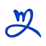# 2022年JS开发提升效率的写法dearweb 发布：2022-09-05 21:51:43阅读：

### 判断空和未定义

```let username;
if (name !== null || name !== undefined || name !== '') {
} else {
}```

`let userName = name || "";`

### 数组定义

```let a = new Array();
a = "s1";
a = "s2";
a = "s3";```

`let a = ["s1", "s2", "s3"]`

### 三元运算符

```let big;
if (x > 10) {
big = true;
}
else {
big = false;
}```

`let big = x > 10 ? true : false;`

`let big = x > 10;`

```function x() { console.log('x') };
function y() { console.log('y') };

let z = 3;
if (z == 3) {
x();
} else {
y();
}```

```function x() { console.log('x') };
function y() { console.log('y') };

let z = 3;
(z==3 ? x : y)(); // Shortcut```

`if (likeJs === true)`

`if (likeJs)`

### 声明变量

```let x;
let y;
let z = 3;```

`let x, y, z = 3;`

### 使用正则表达式

```https://developer.mozilla.org/enUS/docs/Web/JavaScript/Guide/Regular_Expressions

https://regexr.com/

https://regex101.com/```

#### charAt() 快捷键

`"string".charAt(0);`

`"string"; // Returns 's'`

### 以 10 为底的幂

`for (let i = 0; i < 1e4; i++) {`

### 模板文字

`const question = “My number is “ + number + “, ok?”`

`const question = `My number is \${number}, ok?``

### 箭头函数

```function sum(n1,n2){
return n1 + n2;
}```

```const sum = function(n1,n2){
return n1+n2;
}```

`const sum = (n1,n2) => n1 + n2;`

### 参数解构

```function init(config){
const s = config.s;
const t = config.t;
return s + t;// or config.s + config.t
}

init({s: "1", t: "2"});```

```function init({s, t}){
return s + t;
}

init({s: 1, t: 2});```

```function init({s = 10, t = 20}){
return s + t;
}
init({s: 1});```

### 键值名称

```const name = "Joseph"
const person = { name: name }
// { name: "Joseph" }```

```const name = "Joseph"
const person = { name }
// { name: "Joseph" }```

```const name = "Joseph"
const canCode = true
const person = { name, canCode }
// { name: "Joseph", canCode: true }```

### Map

```const animals = [
{
"name": "cat",
"size": "small",
"weight": 5
},
{
"name": "dog",
"size": "small",
"weight": 10
},
{
"name": "lion",
"size": "medium",
"weight": 150
},
{
"name": "elefante",
"size": "large",
"weight": 5000
}
]```

```let animalNames = [];

for (let i = 0; i < animals.length; i++) {
animalNames.push(animals[i].name);
}```

```let animalNames = animais.map((animal) => {
return animal.nome;
})```

### Filter

```let smallAnimals = [];

for (let i = 0; i < animals.length; i ++) {
if (animals[i].size === "small") {
smallAnimals.push(animals[i])
}
}```

```let smallAnimals = animais.filter((animal) => {
return animal.size === "small"
})```

Filter 期望一个函数的参数是当前迭代的对象（如在 foreach 中），它应该返回一个布尔值，指示该对象是否将成为返回数组的一部分（true 表示它通过了测试 并将成为其中的一部分）。

### Reduce

Javascript 的另一个重要特性是 reduce。它允许我们以非常简单和强大的方式在集合之上进行分组和计算。例如，如果我们想将动物对象数组中所有动物的重量相加，我们会怎么做？

```let totalWeight = 0;

for (let i = 0; i < animals.length; i++) {
totalWeight += animals[i].weight;
}```

```let totalWeight = animals.reduce((total, animal) => {
return total += animal.weight;
}, 0)```

Reduce 需要一个带有以下参数的函数：

24人点赞 返回栏目 提问